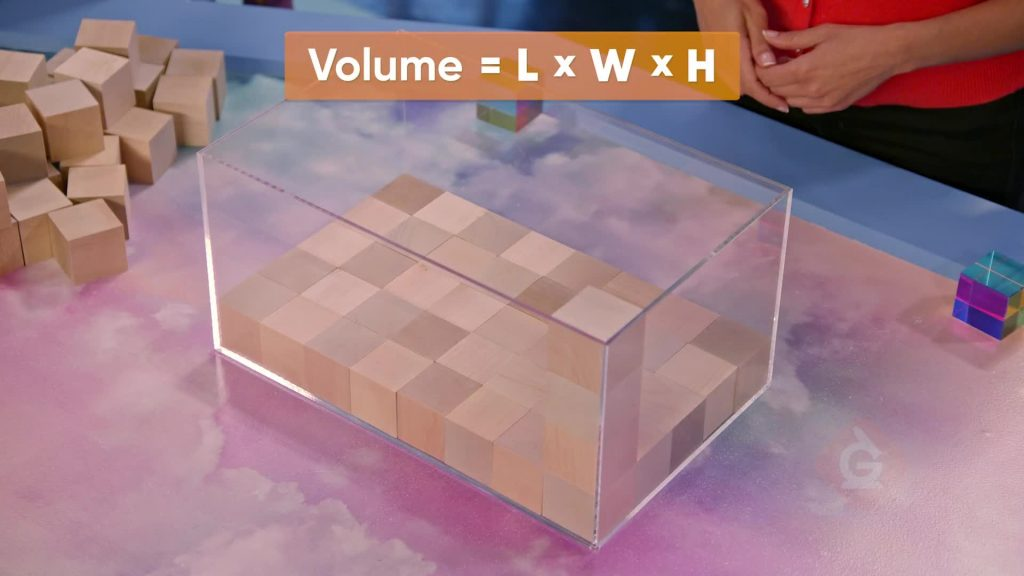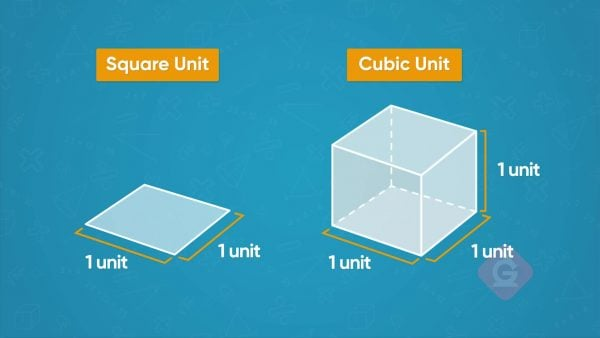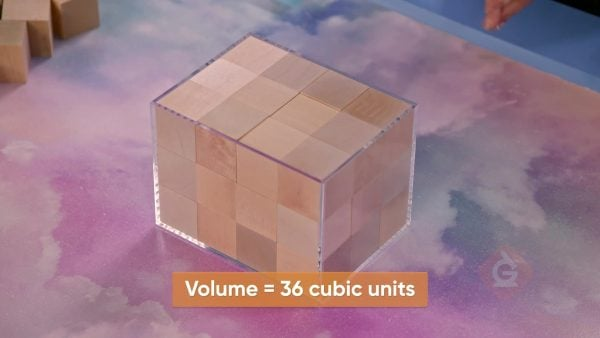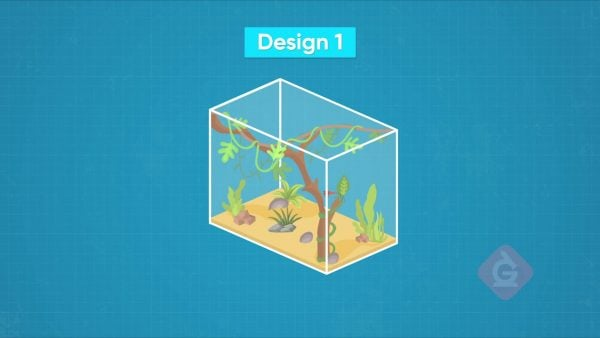Calculate Volume with V = l×w×h | Science Lesson For Kids | Grades 3-5
1%
It was processed successfully!WHAT IS CALCULATING VOLUME (l x w x h)?

Students learn to calculate the volume of a rectangular prism using the formula . They also calculate the volume of composite figures that are made up of two or more rectangular prisms.

To better understand calculating volume…

WHAT IS CALCULATING VOLUME (l x w x h)?. Students learn to calculate the volume of a rectangular prism using the formula . They also calculate the volume of composite figures that are made up of two or more rectangular prisms. To better understand calculating volume…

## LET’S BREAK IT DOWN!

### Cubic UnitsLet’s say you have a box. You have smaller cubes that measure 1 unit by 1 unit by 1 unit. We call each one of these cubes 1 cubic unit. You find that exactly 16 cubic units fit in the box. We can use this as a measure of the volume of the box. Volume is the amount of space inside a 3-dimensional object. We can say the volume of the box is 16 cubic units. Try this one yourself. You have a box that can fit exactly 20 cubes that each measure 1 inch by 1 inch by 1 inch. What is the volume of the box?

Cubic Units Let’s say you have a box. You have smaller cubes that measure 1 unit by 1 unit by 1 unit. We call each one of these cubes 1 cubic unit. You find that exactly 16 cubic units fit in the box. We can use this as a measure of the volume of the box. Volume is the amount of space inside a 3-dimensional object. We can say the volume of the box is 16 cubic units. Try this one yourself. You have a box that can fit exactly 20 cubes that each measure 1 inch by 1 inch by 1 inch. What is the volume of the box?

### Packing ToysLet’s say you want to pack cube-shaped toys into a box for shipping. Each cube-shaped toy measures 1 unit on each side. We’ll call that 1 cubic unit. The base layer of the shipping container is 3 units by 4 units. You find you can fit 12 cubes in the base. Then you find you can fit a second layer of 12 cubes. When you put in a third layer of 12 cubes, the box is filled. When you add the number of cubes in each layer you get 36 cubic units. This is the volume of the box. When the third layer is added we see that the dimensions of the box are 3 units by 4 units by 3 units. We can also find the volume by multiplying 3 units by 4 units by 3 units to get 36 cubic units. The formula for a rectangular prism like the box is V = l×w×h. Try this one yourself. You have a box that measures 2 units by 5 units by 7 units. What is the volume of the box?

Packing Toys Let’s say you want to pack cube-shaped toys into a box for shipping. Each cube-shaped toy measures 1 unit on each side. We’ll call that 1 cubic unit. The base layer of the shipping container is 3 units by 4 units. You find you can fit 12 cubes in the base. Then you find you can fit a second layer of 12 cubes. When you put in a third layer of 12 cubes, the box is filled. When you add the number of cubes in each layer you get 36 cubic units. This is the volume of the box. When the third layer is added we see that the dimensions of the box are 3 units by 4 units by 3 units. We can also find the volume by multiplying 3 units by 4 units by 3 units to get 36 cubic units. The formula for a rectangular prism like the box is V = l×w×h. Try this one yourself. You have a box that measures 2 units by 5 units by 7 units. What is the volume of the box?

### Fish TankLet’s say you want to get a fish tank that has a volume of at least 20,000 cubic centimeters. You find a fish tank that measures 50 centimeters by 30 centimeters by 20 centimeters. You multiply the three dimensions: 50 × 30 × 20 = 30,000 cubic centimeters. Since 30,000 cubic centimeters is greater than 20,000 cubic centimeters, you know this tank is big enough for your fish. Try this one yourself. You want to buy a fish tank that has a volume of at least 20,000 cubic centimeters for your friend. You find a fish tank that measures 30 centimeters by 30 centimeters by 40 centimeters. Is this fish tank big enough?

Fish Tank Let’s say you want to get a fish tank that has a volume of at least 20,000 cubic centimeters. You find a fish tank that measures 50 centimeters by 30 centimeters by 20 centimeters. You multiply the three dimensions: 50 × 30 × 20 = 30,000 cubic centimeters. Since 30,000 cubic centimeters is greater than 20,000 cubic centimeters, you know this tank is big enough for your fish. Try this one yourself. You want to buy a fish tank that has a volume of at least 20,000 cubic centimeters for your friend. You find a fish tank that measures 30 centimeters by 30 centimeters by 40 centimeters. Is this fish tank big enough?

### Sugar GliderLet’s say you have a sugar glider and you want to get an enclosure for it. You see an interesting enclosure at the pet store that looks like it is two rectangular prisms put together to make an L shape. You want to find the volume of the enclosure. If you find the volume of each rectangular prism, you can add the two volumes together to get the volume of the enclosure. One prism measures 4 feet by 2 feet by 3 feet. To find the volume of that rectangular prism you multiply 4 × 2 = 8 and 8 × 3 = 24. The volume of this rectangular prism is 24 cubic feet. The other prism measures 4 feet by 2 feet by 2 feet. To find the volume of that prism you multiply 4 × 2 = 8 and 8 × 2 = 16. The volume of this rectangular prism is 16 cubic feet. To find the volume of the enclosure, you add 24 + 16 = 40. The volume of the enclosure is 40 cubic feet. Try this one yourself. You want to find the volume of an enclosure for a sugar glider. The enclosure is made up of two rectangular prisms. One rectangular prism measures 3 feet by 6 feet by 2 feet. The other prism measures 4 feet by 2 feet by 4 feet. What is the volume of this enclosure?

Sugar Glider Let’s say you have a sugar glider and you want to get an enclosure for it. You see an interesting enclosure at the pet store that looks like it is two rectangular prisms put together to make an L shape. You want to find the volume of the enclosure. If you find the volume of each rectangular prism, you can add the two volumes together to get the volume of the enclosure. One prism measures 4 feet by 2 feet by 3 feet. To find the volume of that rectangular prism you multiply 4 × 2 = 8 and 8 × 3 = 24. The volume of this rectangular prism is 24 cubic feet. The other prism measures 4 feet by 2 feet by 2 feet. To find the volume of that prism you multiply 4 × 2 = 8 and 8 × 2 = 16. The volume of this rectangular prism is 16 cubic feet. To find the volume of the enclosure, you add 24 + 16 = 40. The volume of the enclosure is 40 cubic feet. Try this one yourself. You want to find the volume of an enclosure for a sugar glider. The enclosure is made up of two rectangular prisms. One rectangular prism measures 3 feet by 6 feet by 2 feet. The other prism measures 4 feet by 2 feet by 4 feet. What is the volume of this enclosure?

## CALCULATE VOLUME (l x w x h) VOCABULARY

Volume
The amount of space inside a 3-dimensional object.
Formula
A rule written with mathematical symbols. V = l×w×h is a formula.
Rectangular Prism
A 3-dimensional solid shape that has 6 faces.
Length
A measure of how long an object is.
Width
A measure of how wide an object is.
Height
A measure of how tall an object is.
Used to measure; for example: inch, square foot, cubic meter.
Used to measure, a square that is 1 unit long and 1 unit wide.
Used to measure, a cube that is 1 unit long, 1 unit wide, and 1 unit tall.

## CALCULATE VOLUME (l x w x h) DISCUSSION QUESTIONS

### What is the volume of a rectangular prism that has length 3 feet, width 2 feet and height 5 feet? Explain your process.

The volume is 30 cubic feet. I multiplied 3×2×5.

### Why do you use cubic units to measure volume?

When I find volume, I find the number of unit cubes required to fill the object. The units that I use to measure are unit cubes.

### Mio says that the volume of a box in the shape of a rectangular prism that measures 4 cm by 5 cm by 6 cm is 15 cm. Is she correct? Explain.

Mio is not correct. The volume of the box is 120 cubic cm. I think Mio added the measurements instead of multiplying, and used units instead of cubic units.

### Write a word problem where you need to find the volume of a rectangular prism to find the answer.

Roberto has a fish tank in the shape of a rectangular prism that measures 2 feet by 3 feet by 2 feet. What is the volume of the fish tank?

### What is the volume of a figure that is made up of two rectangular prisms? One rectangular prism has these dimensions: 7 cm by 2 cm by 3 cm. The other rectangular prism has these dimensions: 4 cm by 6 cm by 2 cm. Explain how you found the volume.

One rectangular prism has a volume of 7 × 2 × 3 = 42 cubic cm. The other rectangular prism has a volume of 4 × 6 × 2 = 48 cubic cm. I added 42 cubic cm and 48 cubic cm and found that the volume of the figure is 90 cubic cm.
X

## Success

We’ve sent you an email with instructions how to reset your password.
Ok